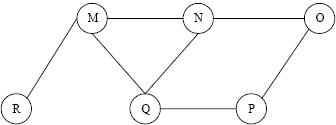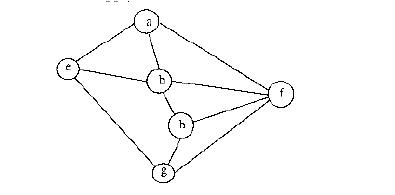Courses

# Test: Graph Based Algorithms- 1

## 15 Questions MCQ Test Algorithms | Test: Graph Based Algorithms- 1

Description
This mock test of Test: Graph Based Algorithms- 1 for Computer Science Engineering (CSE) helps you for every Computer Science Engineering (CSE) entrance exam. This contains 15 Multiple Choice Questions for Computer Science Engineering (CSE) Test: Graph Based Algorithms- 1 (mcq) to study with solutions a complete question bank. The solved questions answers in this Test: Graph Based Algorithms- 1 quiz give you a good mix of easy questions and tough questions. Computer Science Engineering (CSE) students definitely take this Test: Graph Based Algorithms- 1 exercise for a better result in the exam. You can find other Test: Graph Based Algorithms- 1 extra questions, long questions & short questions for Computer Science Engineering (CSE) on EduRev as well by searching above.
QUESTION: 1

### Which of the following is an advantage of adjacency list representation over adjacency matrix representation of a graph?

Solution:

An array of lists is used. The size of the array is equal to the number of vertices. Let the array be an array[]. An entry array[i] represents the list of vertices adjacent to the ith vertex. This representation can also be used to represent a weighted graph. The weights of edges can be represented as lists of pairs.

QUESTION: 2

### What are the appropriate data structures for following algorithms? 1) Breadth First Search                            2) Depth First Search                             3) Prim's Minimum Spanning Tree                  4) Kruskal' Minimum Spanning Tree

Solution:

1) Breadth First Search uses Queue

2) Depth First Search uses Stack

3) Prim's Minimum Spanning Tree uses Priority Queue.

4) Kruskal' Minimum Spanning Tree uses Union Find.

QUESTION: 3

### The degree sequence of a simple graph is the sequence of the degrees of the nodes in the graph in decreasing order. Which of the following sequences can not be the degree sequence of any graph? I. 7, 6, 5, 4, 4, 3, 2, 1 II. 6, 6, 6, 6, 3, 3, 2, 2 III. 7, 6, 6, 4, 4, 3, 2, 2 IV. 8, 7, 7, 6, 4, 2, 1, 1

Solution:

In sequence IV, we have a vertex with degree 8 which is not possible in a simple graph (no self loops and no multiple edges) with total vertex count as 8. Maximum possible degree in such a graph is 7.

In sequence II, four vertices are connected to 6 other vertices, but remaining 4 vertices have degrees as 3, 3, 2 and 2 which are not possible in a simple graph (no self loops and no multiple edges).

QUESTION: 4

The Breadth First Search algorithm has been implemented using the queue data structure. One possible order of visiting the nodes of the following graph isSolution:

Option (A) is MNOPQR. It cannot be a BFS as the traversal starts with M, but O is visited before N and Q. In BFS all adjacent must be visited before adjacent of adjacent. Option (B) is NQMPOR. It also cannot be BFS, because here, P is visited before O. (C) and (D) match up to QMNP. We see that M was added to the queue before N and P (because M comes before NP in QMNP). Because R is M's neighbor, it gets added to the queue before the neighbor of N and P (which is O). Thus, R is visited before O.

QUESTION: 5

The most efficient algorithm for finding the number of connected components in an undirected graph on n vertices and m edges has time complexity. (A) θ(n) (B) θ(m) (C) θ(m + n) (D) θ(mn)

Solution:

Connected components can be found in O(m + n) using Tarjan's algorithm. Once we have connected components, we can count them.

QUESTION: 6

Let G be an undirected graph. Consider a depth-first traversal of G, and let T be the resulting depth-first search tree. Let u be a vertex in G and let v be the first new (unvisited) vertex visited after visiting u in the traversal. Which of the following statements is always true?

Solution:

In DFS, if 'v' is visited
after 'u', then one of the following is true.
1) (u, v) is an edge.
u
/   \
v     w
/     / \
x     y   z

2) 'u' is a leaf node.
w
/   \
x     v
/     / \
u     y   z
In DFS, after visiting a node, we first recur for all unvisited children. If there are no unvisited children (u is leaf), then control goes back to parent and parent then visits next unvisited children.

QUESTION: 7

Consider an undirected unweighted graph G. Let a breadth-first traversal of G be done starting from a node r. Let d(r, u) and d(r, v) be the lengths of the shortest paths from r to u and v respectively, in G. lf u is visited before v during the breadth-first traversal, which of the following statements is correct?

Solution:

d(r, u) and d(r, v) will be equal when u and v are at same level, otherwise d(r, u) will be less than d(r, v)

QUESTION: 8

How many undirected graphs (not necessarily connected) can be constructed out of a given set V= {V 1, V 2,…V n} of n vertices ?

Solution:

In an undirected graph, there can be maximum n(n-1)/2 edges. We can choose to have (or not have) any of the n(n-1)/2 edges. So, total number of undirected graphs with n vertices is 2^(n(n-1)/2).

QUESTION: 9

Consider the following graph,Among the following sequences:
(I) a b e g h f
(II) a b f e h g
(III) a b f h g e
(IV) a f g h b e
Which are depth first traversals of the above graph?

Solution:

We can check all DFSs for following properties.
In DFS, if a vertex 'v' is visited
after 'u', then one of the following is true.
1) (u, v) is an edge.
u
/   \
v     w
/     / \
x     y   z

2) 'u' is a leaf node.
w
/   \
x     v
/     / \
u     y   z
In DFS, after visiting a node, we first recur for all unvisited children. If there are no unvisited children (u is leaf), then control goes back to parent and parent then visits next unvisited children.

QUESTION: 10

Which of the following statements is/are TRUE for an undirected graph? P: Number of odd degree vertices is even Q: Sum of degrees of all vertices is even

Solution:

P is true for undirected graph as adding an edge always increases degree of two vertices by 1. Q is true: If we consider sum of degrees and subtract all even degrees, we get an even number because every edge increases the sum of degrees by 2. So total number of odd degree vertices must be even.

QUESTION: 11

Make is a utility that automatically builds executable programs and libraries from source code by reading files called makefiles which specify how to derive the target program. Which of the following standard graph algorithms is used by Make.

Solution:

Make can decide the order of building a software using topological sorting. Topological sorting produces the order considering all dependencies provide by makefile. See following for details. Topological Sorting

QUESTION: 12

Given two vertices in a graph s and t, which of the two traversals (BFS and DFS) can be used to find if there is path from s to t?

Solution:

We can use both traversals to find if there is a path from s to t.

QUESTION: 13

Consider an undirected random graph of eight vertices. The probability that there is an edge between a pair of vertices is 1/2. What is the expected number of unordered cycles of length three?

Solution:

A cycle of length 3 can be formed with 3 vertices. There can be total 8C3 ways to pick 3 vertices from 8. The probability that there is an edge between two vertices is 1/2. So expected number of unordered cycles of length 3 = (8C3)*(1/2)^3 = 7

QUESTION: 14

Is following statement true/false? A DFS of a directed graph always produces the same number of tree edges, i.e., independent of the order in which vertices are considered for DFS.

Solution:

Consider the following graph. If we start from 'a', then there is one tree edge. If we start from 'b', then there is no tree edge. a→b

QUESTION: 15

Given an undirected graph G with V vertices and E edges, the sum of the degrees of all vertices is

Solution:

Since the given graph is undirected, every edge contributes as 2 to sum of degrees. So the sum of degrees is 2E.